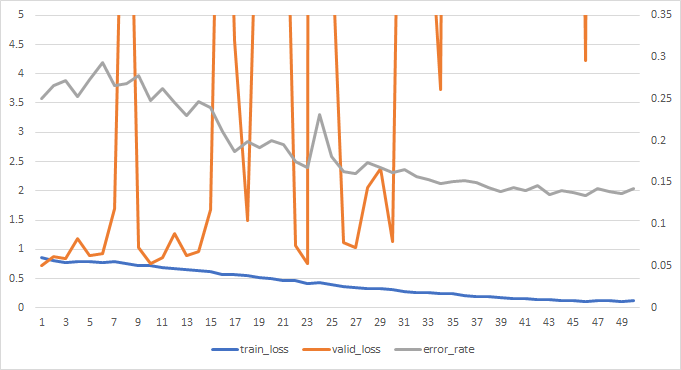# Got trouble in training cifar10

I had tried a standard method to train resnet18 for cifar10. The codes are below.

``````import matplotlib
matplotlib.use('svg')
import matplotlib.pyplot as plt

import numpy as np
from fastai import  *
from models import *
import pandas as pd
from fastai.vision import *
path =untar_data(URLs.CIFAR, 'data/data11752/cifar10.tgz')
from fastai.torch_core import defaults
print('device',defaults.device)

databunch=(ImageDataBunch.from_folder(path,valid='test',
size=32,
ds_tfms=get_transforms(),
.normalize(([0.4914, 0.4822, 0.4465],[0.2023, 0.1994, 0.2010])))

learner = cnn_learner(databunch,models.resnet18,path='work',metrics=error_rate,callback_fns=[callbacks.CSVLogger])
learner.fit_one_cycle(1,0.1,wd=5e-4)
learner.unfreeze()
learner.fit_one_cycle(50,0.01,wd=5e-4)
learner.save('stage1')
``````

With the help of ClassificationInterpretation, I found automobile is classified as cat.The valid loss is extremely high. It seems like the model is overfitting.
What caused this? And how to solve it?

maybe because you override the default weight decay `wd=1e-2`. Try a higher weight decay for regularization.